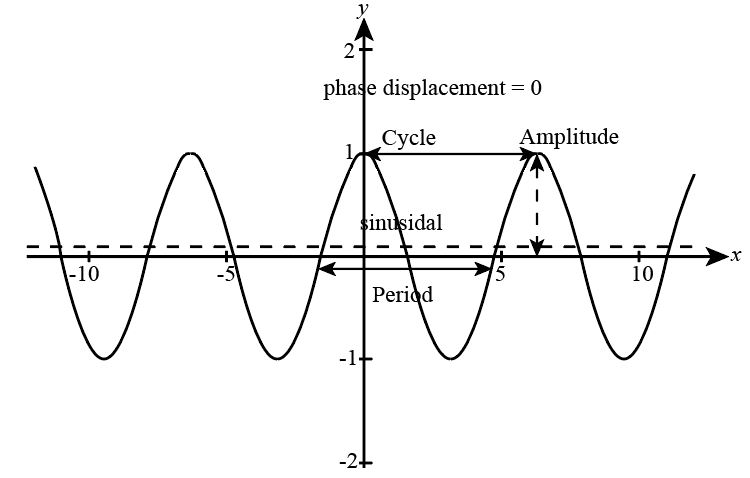×
Get Full Access to Precalculus With Trigonometry: Concepts And Applications - 1 Edition - Chapter 3-8 - Problem R.1
Get Full Access to Precalculus With Trigonometry: Concepts And Applications - 1 Edition - Chapter 3-8 - Problem R.1

×

# a. Sketch the graph of a sinusoid. On the graph, show the difference in meaning betweenISBN: 9781559533911 468

## Solution for problem R.1 Chapter 3-8

Precalculus with Trigonometry: Concepts and Applications | 1st Edition

• Textbook Solutions
• 2901 Step-by-step solutions solved by professors and subject experts
• Get 24/7 help from StudySoup virtual teaching assistantsPrecalculus with Trigonometry: Concepts and Applications | 1st Edition

4 5 1 412 Reviews
14
2
Problem R.1

a. Sketch the graph of a sinusoid. On the graph, show the difference in meaning between a cycle and a period. Show the amplitude, the phase displacement, and the sinusoidal axis. b. In y = 3 + 4 cos 5( 10), what name is given to the quantity 5( 10)? R

Step-by-Step Solution:

Step 1 of 3

a) The figure of the sinusoidal function with amplitude, the phase displacement, and the sinusoidal axis and the difference between cycle and period is shown below:Step 2 of 3

Step 3 of 3

##### ISBN: 9781559533911

Precalculus with Trigonometry: Concepts and Applications was written by and is associated to the ISBN: 9781559533911. This full solution covers the following key subjects: . This expansive textbook survival guide covers 106 chapters, and 2321 solutions. Since the solution to R.1 from 3-8 chapter was answered, more than 236 students have viewed the full step-by-step answer. This textbook survival guide was created for the textbook: Precalculus with Trigonometry: Concepts and Applications, edition: 1. The answer to “a. Sketch the graph of a sinusoid. On the graph, show the difference in meaning between a cycle and a period. Show the amplitude, the phase displacement, and the sinusoidal axis. b. In y = 3 + 4 cos 5( 10), what name is given to the quantity 5( 10)? R” is broken down into a number of easy to follow steps, and 51 words. The full step-by-step solution to problem: R.1 from chapter: 3-8 was answered by , our top Calculus solution expert on 03/16/18, 04:16PM.

Unlock Textbook Solution